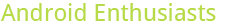## Participation value ratios

8

(click on this box to dismiss)Q&A for enthusiasts and power users of the Android operating system

``````with Generated as (

select ParentId as GenId, sum (Score) as Score
from Posts
where PostTypeId = 2
group by ParentId
), VoteGen as (
select PostId,
sum (case when VoteTypeId = 2 then 1 else 0 end) as GotUp,
sum (case when VoteTypeId = 3 then 1 else 0 end) as GotDown
where VoteTypeId in (2, 3)
group by PostId
), Data as (

select Users.Id as UserId,
DisplayName,
Reputation,
sum (case when AcceptedAnswerId is null then 0 else 1 end) as Accepts,
sum (case when PostTypeId = 1 then 1 else 0 end) as QuestionsAsked,
sum (case when PostTypeId = 2 then 1 else 0 end) as AnswersGiven,
sum (IsNull(VoteGen.GotUp, 0)) as GotUp,
sum (IsNull(VoteGen.GotDown, 0)) as GotDown,
sum (IsNull(Generated.Score, 0)) as Generated
from Posts
left outer join VoteGen on VoteGen.PostId = Posts.Id
left outer join Generated on Posts.Id = Generated.GenId
where Posts.PostTypeId in (1, 2)
group by Users.Id,
Users.DisplayName,
Users.Reputation,

)

select RANK ( ) OVER ( order by ((GiveUp + GiveDown + Accepts) / (1.0 * AnswersGiven + QuestionsAsked + AnswerReplies)) DESC ) as RankFeedBack,
RANK ( ) OVER ( order by ((GiveUp - GiveDown) + Generated) DESC ) as RankValueAdd,
UserId  as [User Link], DisplayName as Name,
Reputation as Rep,

-- Generated -> how much score has been spent as a result of your work
(GiveUp - GiveDown) + Generated as ValueAdd,
Generated,

-- How much feedback you gave as a proportion of your feedback expectations
-- expected to vote on questions you answer
Cast((GiveUp + GiveDown + Accepts) / (1.0 * AnswersGiven + QuestionsAsked + AnswerReplies) as Numeric(10,2)) as FeedbackRatio,

then 1.0
else Accepts / Cast(QuestionsAsked as NUMERIC(10,2))
end as Numeric (10,3)) as AccRat,

then 1.0
else Generated / Cast(QuestionsAsked as NUMERIC(10,2))
end as Numeric (10,3)) as GenRat,

GiveUp as [V+Out],
GiveDown as [v-Out],
GotUp as [V+In],
GotDown as [V-In],
Accepts as [Acc]

--,Hold tight while we fetch your results